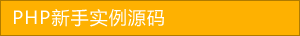﻿ use关键字在php中的使用（含代码） - php应用 - PHP粉丝网

# use关键字在php中的使用（含代码）

##### 发布:smiling　来源: PHP粉丝网 　添加日期：2022-06-23 08:42:54　浏览: 评论：0

use关键字在php中的使用

1、use最常用在给类取别名，还可以用在闭包函数中，代码如下：

1. <?php
2.
3. function test() {
4.
5.     \$a = 'hello'
6.
7.     return function (\$a)use(\$a) {
8.
9.         echo \$a . \$a
10.
11.     };
12.
13.
14. \$b = test();
15.
16. \$b('world');//结果是hellohello

2、use中的参数也可以使用引用传递的，代码如下

1. <?php
2.
3. function test() {
4.
5.     \$a=18;
6.
7.     \$b="Ly"
8.
9.     \$fun = function(\$num\$nameuse(&\$a, &\$b) {
10.
11.         \$a = \$num
12.
13.         \$b = \$name
14.
15.     };
16.
17.     echo "\$b:\$a<br/>"
18.
19.     \$fun(30,'wq');
20.
21.     echo "\$b:\$a<br/>"
22.
23.
24. test();
25.
26. //结果是Ly:18
27.
28. //结果是wq:30

1. <?php
2.
3. function index() {
4.
5. \$a = 1;
6.
7. return function () use(&\$a){
8.
9. echo \$a
10.
11. \$a++;
12.
13. };
14.
15.
16. \$a = index();
17.
18. \$a();
19.
20. \$a();
21.
22. \$a();
23.
24. \$a();
25.
26. \$a();
27.
28. \$a();
29.
30. //123456
31.
32. ?>

## 相关文章